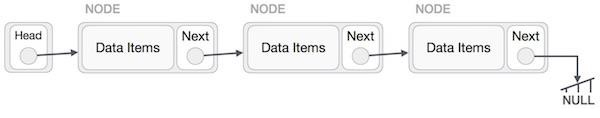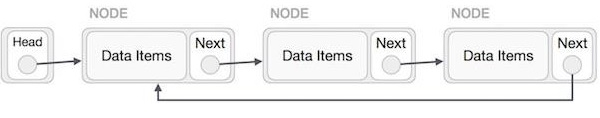# Sum of the nodes of a Circular Linked List in C++

In this problem, we are given a circular linked list. Our task is to create a program to find the sum of the nodes of a Circular Linked List.

We simply need to add all the node values of the linked list.

Some important definitions

• Linked List is a sequence of data structures, which are connected together via links.• Circular Linked List is a variation of the Linked list in which the first element points to the last element and the last element points to the first element. Both Singly Linked List and Doubly Linked List can be made into a circular linked list.Now, let’s take an example to understand the problem,

Input

14 -> 1 -> 7 -> 9 -> 2 -> 6

Output

39

Explanation

sum = 14 + 1 + 7 + 9 + 2 + 6 = 39

To solve this problem, we will traverse the linked list. And add each node’s value to a sum variable. Then return the sum, when the whole list is traversed.

## Algorithm

Step 1 − Initialize sum = 0 and sumPointer =

Step 2 − Do-while sumPointer != head. Do

Step 2.1 − Add current node’s value to sum, i.e. sum += sumPointer → value.

Step 2.2 − Increment pointer to next node, i.e. sumPointer = sumPointer → next.

Step 3 − Return Sum.

## Example

Program to illustrate the solution,

Live Demo

#include <iostream>
using namespace std;
struct Node {
int data;
struct Node* next;
};
void pushNode(struct Node** head_ref, int data) {
struct Node* ptr1 = (struct Node*)malloc(sizeof(struct Node));
ptr1->data = data;
temp = temp->next;
temp->next = ptr1;
}
else
ptr1->next = ptr1;
}
int sum = 0;
do {
sumPointer = sumPointer->next;
sum += sumPointer->data;
}
}
return sum;
}
int main(){
}
The sum of Circular linked list is 39